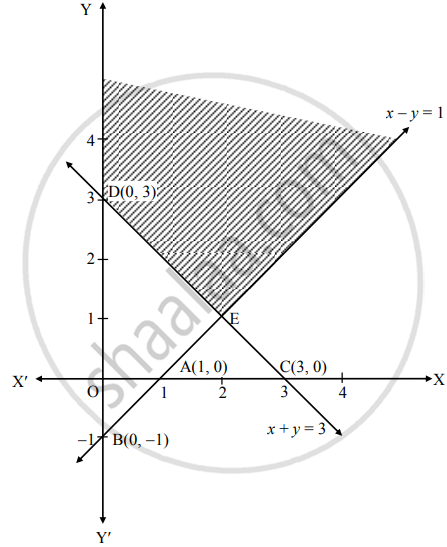# Solve the following problem : Minimize Z = 2x + 3y Subject to x – y ≤ 1, x + y ≥ 3, x ≥ 0, y ≥ 0 - Mathematics and Statistics

Graph

Solve the following problem :

Minimize Z = 2x + 3y Subject to x – y ≤ 1, x + y ≥ 3, x ≥ 0, y ≥ 0

#### Solution

To find the graphical solution, construct the table as follows:

 Inequation Equation Double intercept form Points (x1, x2) Region x – y ≤ 1 x – y = 1 x/(1) + y/(-1) = 1 A (1, 0)B (0, –1) 0 – 0 ≤ 1∴ 0 ≤ 1∴ Origin-side x + y ≥ 1 x + y = 3 x/(3) + y/(3) = 1 C (3, 0)D (0, 3) 0 + 0 ≥ 3∴ 0 ≥ 3∴ non-origin side x ≥ 0 x = 0 – R.H.S. of Y- axis y ≥ y = 0 above X-axisThe shaded portion Y' DE is the feasible region.
Whose vertices are D(0, 3) and E
E is the point of intersection of lines
x – y = 1          …(i)
x + y = 3         …(ii)
∴ By (i) + (ii), we get
x – y = 1
x + y = 3
2x      = 4

∴ x = (4)/(2) = 2
Substituting x = 2 in (i), we get
2 – y = 1
∴ y  = 1
∴ E(2, 1)
Here, the objective function is Z = 2x + 3y
Now, we will find minimum value of Z as follows:

 Feasible points The value of Z = 2x + 3y D(0, 3) Z = 2(0) + 3(3) = 9 E(2, 1) Z = 2(2) + 3(1) = 4 + 3 = 7

∴ Z has minimum value 7 at E(2, 1)
∴ Z is minimum, when x = 2, y = 1.

Concept: Mathematical Formulation of Linear Programming Problem
Is there an error in this question or solution?

#### APPEARS IN

Balbharati Mathematics and Statistics 2 (Commerce) 12th Standard HSC Maharashtra State Board
Chapter 6 Linear Programming
Miscellaneous Exercise 6 | Q 4.04 | Page 104